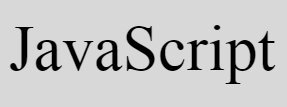# JavaScript Programming Examples

« JavaScript Tutorial Next Program »

Right now, JavaScript is most demanded programming language in the world. JavaScript is also the most popular and widely used programming language all over.

JavaScript is a language of Web programming. So to program on the web, JavaScript is essentially required.

Therefore, we're providing a lot of JavaScript programs to practice and learn. Each and every JavaScript program is provided with its static and dynamic output.

Static output is the output, shown through pictorial representation while executing the program. That is, at the time of testing and executing, snapshot of each and every output was taken and provided over here to clear your doubt on any JavaScript program.

Whereas dynamic output means, the live output of every program is also provided. Live output means, you can check the program with your input also. You'll understand the things what I mean to say, later on.

Note - Here, static and dynamic output doesn't relates to technical word, it is just a remind that how these two output is, in this series of JavaScript programs.

Note - Here, each and every JavaScript program is well tested.

There are too many programs is created through JavaScript. So it is not possible to attach all the program in one article, so we've created the series of articles on JavaScript programs.

Every JavaScript program is created with very simple code initially, so that beginners can also understand all the programs available here. After simple code for beginner, a little complicated code is also given in each and every topic.

## JavaScript Programs for Beginners

In each and every JavaScript program, we've provided simplest JavaScript code to do the task. So that, a beginner JavaScript learner can also understand the topic. Later on, input from user also gets received and calculation gets performed based on user input.

For example, if user wants to learn how to add two numbers in JavaScript, then a simple JavaScript code like:

```var a=3, b=7, sum;
sum = a+b;```

is given before the actual program that receives input from user and prints the result and all those type of programs.

In above JavaScript two lines of code, the first line declares three variables, a, b and sum. Values 3 and 7 is already initialized to first two variables. Whereas in second line, the value, a+b or 3+7 or 10 gets initialized to sum.

#### Top JavaScript Programs

Here are the list of some popular programs in JavaScript:

## JavaScript Program Examples with Output

Every JavaScript program written in this series, is well tested with its output. For example, let's have a look at the following JavaScript program to check whether the number is less than 10 or not:

```var num=12;
if(num<10)
document.write(num + " is less than 10");
else
document.write(num + " is not less than 10");```

This is the simplest JavaScript code, shows the demo that how the simplest JavaScript code is created in each and every article for beginner learner. And here is the same JavaScript program written with HTML:

```<!doctype html>
<html>
<script>
var num=12;
if(num<10)
document.write(num + " is less than 10");
else
document.write(num + " is not less than 10");
</script>
<body>

</body>
</html>```

Save the above code in a file with .html extension say codescracker.html. Now open this file in a web browser. Here is the output you will see. This is what static output means, for this series of JavaScript programs:

Now change the value of num with 8 and save the code in same file. Therefore after refreshing the web browser, here is the output you will see this time:

Note - The document.write() method writes data inside its braces () to an HTML output.

Finally this is the actual program that receives input from user, then JavaScript code gets in action based on user input:

```<!doctype html>
<html>
<script>
function jsFun()
{
var val, elem;
val = parseInt(document.getElementById("num").value);
if(val)
{
if(val<10)
document.getElementById("paraTwo").innerHTML = "Less than 10";
else
document.getElementById("paraTwo").innerHTML = "Greater than 10";
}
}
</script>
<body>

<p id="paraOne">Enter a Number: <input id="num">
<button onclick="jsFun()">Enter</button></p>
<p id="paraTwo"></p>

</body>
</html>```

Here is its sample output:

Now enter a number say 19 and click on the Enter button to see the following output:

#### Live Output of Previous JavaScript Program

And here is the live output. This is what dynamic output means for this series of JavaScript programs:

Enter a Number:

When user clicks on the button, Enter, a function named jsFun() gets called. And all the statements of this function gets executed. That is, two variables val and elem gets declared, and the statement:

`val = parseInt(document.getElementById("num").value);`

states that, an int (integer) value of an HTML element whose id is num gets initialized to val variable. Now the code,
`if(val)`, checks whether val has some value or it is empty. This code is created to check whether user enters some data then clicks on Enter button, or clicked on Enter button without providing the input.

Now if user enters the number say 19, then val=19. Now using if, we've checked whether the value of val (19) is less than 10 or not. Because the condition, val<10 or 19<10 evaluates to be false, therefore program flow does not goes inside the if's body, rather it goes to its else's part, and the statement:

`document.getElementById("paraTwo").innerHTML = "Greater than 10";`

gets executed, which states that, the value of an HTML element whose id is paraTwo gets printed with Greater than 10.

Note - From next article (chapter), you will learn each and every block of code used for JavaScript program

JavaScript Online Test

« JavaScript Tutorial Next Program »